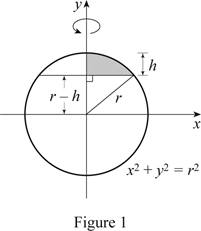# The volume of a segment of a sphere V = 1 3 π h 2 ( 3 r − h ) .### Single Variable Calculus: Concepts...

4th Edition
James Stewart
Publisher: Cengage Learning
ISBN: 9781337687805### Single Variable Calculus: Concepts...

4th Edition
James Stewart
Publisher: Cengage Learning
ISBN: 9781337687805

#### Solutions

Chapter 6, Problem 3P

(a)

To determine

## To show: The volume of a segment of a sphere V=13πh2(3r−h).

Expert Solution

The volume of the segment of a sphere is V=13πh2(3rh)_.

### Explanation of Solution

Given information:

The segment of a sphere with radius r and height h.

Consider that the Equation of circle as x2+y2=r2 (1)

Rearrange Equation (1).

x2=r2y2x=r2y2

The dimensions of the sphere as shown in Figure 1.Refer to Figure 1.

Consider that the sphere is obtained by rotating the circle x2+y2=r2 about y-axis.

The region lies between a=rh and b=r.

Calculation:

The expression to find the volume of the segment of a sphere as shown below:

V=abA(y)dy (2)

Find the area of the segment of a sphere as shown below.

A(y)=πr2=π(r2y2)2=π(r2y2)

Substitute (rh) for a, r for b, and π(r2y2) for A(y) in Equation (2).

V=rhrπ(r2y2)dy=π(r2yy33)rhr=π(r2×rr33(r2(rh)(rh)33))=π(r3r33(rh)(r2(rh)23))

V=π(2r33(rh)3(3r2(r2+h22rh)))=13π(2r3(rh)(2r2+2rhh2))=13π(2r32r32r2h+rh2+2r2h+2rh2h3)=13π(3rh2h3)

=13πh2(3rh)

Therefore, the volume of the segment of a sphere is V=13πh2(3rh)_.

(b)

To determine

### To calculate: The value of x using Newton’s method.

Expert Solution

The value of x by using Newton’s method is 0.2235.

### Explanation of Solution

Given information:

A sphere of radius 1 is sliced by a plane at a distance x from the center.

The volume of one segment is twice the volume of the other.

The Answer of the equation 3x39x+2=0, 0<x<1 is x.

Calculation:

Find the volume of the sphere as shown below.

V=43πr3 (3)

Substitute 1 for r in Equation (3).

V=43π(1)3=43π

Consider the smaller segment has height h=1x.

Refer to part (a).

Volume of the segment of a sphere as shown below:

V=13πh2(3rh) (4)

Substitute 1 for r and 1x for h in Equation (4).

V=13π(1x)2(3×1(1x))=13π(1x)2(x+2)

This volume must be 13 of the total volume of the sphere.

Substitute 43π for V in the above Equation.

13(43π)=13π(1x)2(x+2)43=(1+x22x)(x+2)x+x32x2+2+2x24x=43x33x+2=43

3x39x+6=43x39x+2=0

Apply Newton’s method as shown below.

xn+1=xnf(xn)f(xn) (5)

Consider f(x)=3x39x+2

Differentiate both sides of the Equation.

f(x)=9x29

Substitute 3x39x+2 for f(x) and 9x29 for f(x) in Equation (5).

xn+1=xnxn39xn+23xn29 (6)

Consider x1=0.

Substitute 1 for n in Equation (6).

x2=x1x139x1+23x129=00+209=0.2222

Substitute 2 for n in Equation (6).

x3=x2x239x2+23x229=0.22220.222239×0.2222+23×0.222229=0.2222+0.00130.2235

Substitute 2 for n in Equation (6).

x4=x3x339x3+23x329=0.22350.223539×0.2235+23×0.223529=0.2222+0.00130.2235

x30.2235x4

Therefore, the value of x by using Newton’s method is 0.2235.

(c)

To determine

### To calculate: sinking depth of the sphere.

Expert Solution

The sinking depth of the sphere is 0.6736 m.

### Explanation of Solution

Given information:

Wooden sphere of radius as 0.5 m.

Specific gravity of the wooden sphere is 0.75.

The depth x to which a floating sphere of radius r sinks in water is a root of the equation as follows:

x33rx2+4r3s=0

Calculation:

Show the root of the equation as shown below.

x33rx2+4r3s=0 (7)

Substitute 0.5 m for r and 0.75 for s in Equation (7).

x33(0.5)x2+4(0.5)3×0.75=0x332x2+4×18×34=08x312x2+3=0

Consider f(x)=8x312x2+3

Differentiate both sides of the Equation.

f(x)=24x224x

Apply Newton’s method.

Substitute 8x312x2+3 for f(x) and 24x224x for f(x) in Equation (5).

xn+1=xn8xn312xn2+324xn224 (8)

Consider x1=0.5.

Substitute 1 for n in Equation (8).

x2=x18x1312x12+324x1224=0.58×0.5312×0.52+324×0.5224=0.5+0.0556=0.5556

Substitute 2 for n in Equation (8).

x3=x28x2312x22+324x2224=0.55568×0.5556312×0.55562+324×0.5556224=0.5556+0.0402=0.5958

Substitute 3 for n in Equation (8).

x4=x38x3312x32+324x3224=0.59588×0.5958312×0.59582+324×0.5958224=0.5958+0.0279=0.6237

Approximately the depth should be x=0.6736.

Therefore, the sinking depth of the sphere is 0.6736 m.

(d) i)

To determine

### To calculate: The speed of rising the water level in the bowl from instant of water level at 3 inches deep.

Expert Solution

The speed of rising the water level in the bowl from instant of water level at 3 inches deep is 0.003in/s_

### Explanation of Solution

Given information:

The shape of the bowl is hemisphere.

The radius of hemispherical bowl is 5 inches.

Water is running into the bowl at the rate of 0.2in3/s.

Depth of water is 3 inches.

Calculation:

Show the volume of the segment of a sphere as follows:

V=13πh2(3rh)=πrh213πh3

Differentiate both sides of the Equation with respect to time t.

dVdt=2πrhdhdtπh2dhdt (9)

Find the speed of the water level in the bowl rising at the instant as shown below.

Substitute 5 inches for r, 0.2in3/s for dVdt, and 3 inches for h in Equation (9).

0.2=2π×5×3dhdtπ×32dhdt0.2=(94.24828.274)dhdtdhdt=0.003in/s

Therefore, the speed of rising the water level in the bowl from instant of water level at 3 inches deep is 0.003in/s_

ii)

To determine

### To calculate: The time taken to fill the bowl from 4 inch level of water.

Expert Solution

The time taken to fill the bowl from 4 inch level of water is 6.5min_.

### Explanation of Solution

Given information:

The radius of hemispherical bowl is 5 inches.

Water is running into the bowl at the rate of 0.2in3/s.

Depth of water is 4 inches.

Calculation:

Find the volume of the water required to fill the bowl from the instant that the water is 4 in. depth as follows:

V=12×43πr313πh2(3rh)=23πr3πrh2+13πh3

Substitute 5 inches for r and 4 inches for h in the Equation.

V=23π×53π×5×42+13π×43=23×125π80π+643π=π(250240+64)3=743π

Find the time required to fill the bowl as shown below.

T=VdVdt=743π0.2=387.5sec×1min60sec=6.5min

Therefore, The time taken to fill the bowl from 4 inch level of water is 6.5min_.

### Have a homework question?

Subscribe to bartleby learn! Ask subject matter experts 30 homework questions each month. Plus, you’ll have access to millions of step-by-step textbook answers!